Thank you for visiting nature.com. You are using a browser version with limited support for CSS. To obtain the best experience, we recommend you use a more up to date browser (or turn off compatibility mode in Internet Explorer). In the meantime, to ensure continued support, we are displaying the site without styles and JavaScript.

A two-qubit photonic quantum processor and its application to solving systems of linear equations

Abstract

Large-scale quantum computers will require the ability to apply long sequences of entangling gates to many qubits. In a photonic architecture, where single-qubit gates can be performed easily and precisely, the application of consecutive two-qubit entangling gates has been a significant obstacle. Here, we demonstrate a two-qubit photonic quantum processor that implements two consecutive CNOT gates on the same pair of polarisation-encoded qubits. To demonstrate the flexibility of our system, we implement various instances of the quantum algorithm for solving of systems of linear equations.

Introduction

Quantum computers have attracted tremendous interest because they promise to outperform classical computers at a number of tasks1. The long-term goal is to build a universal quantum processor, which can be programmed to solve arbitrary problems2. Doing so will require decomposing quantum computations into sequences of quantum gates acting on only a few qubits1. In particular, any computation can be decomposed using single-qubit gates and a particular two-qubit entangling gate such as controlled-NOT (CNOT). In linear-optical quantum computing, two-qubit gates are difficult to implement because photons do not interact directly, meaning that the difficulty of a computation can be described by the number of entangling gates it requires.

Entangling gates can be implemented optically by introducing an effective nonlinearity via measurements3, but this implies, in the simplest case, that output photons of a two-qubit gate need to be measured to ensure correct gate operation, making another application of gates to the same pair of qubits impossible4,5,6,7,8,9,10,11. Here, we demonstrate two CNOT gates acting on the same pair of qubits. We implement the two CNOTs differently: the success of the first gate is heralded by the successful detection of two ancillary photons12,13, while the second gate is probabilistic5,6,7. In distinction to previous work11, our scheme does not involve qubit recycling which, in principle, can be performed deterministically.

We then use our setup to determine the solution of several two-dimensional systems of linear equations. Whereas for a classical computer the number of computational steps needed to solve a linear system is at least proportional to the number of variables, a recently developed quantum algorithm could, in some circumstances, make the computational time proportional to only the logarithm of the number of variables14. An important difference is that the quantum algorithm calculates the expectation value of an operator associated with the solution rather than the solution itself. We note that this algorithm has been implemented previously in photonic systems15 and also using NMR techniques16,17. We use the algorithm as an example of the correct operation of two consecutive CNOTs, a flexible approach that differs from previous implementations. As we note below, our method is as powerful as that in15. Our implementation thus presents a general quantum circuit with two consecutive CNOT gates which can be flexibly adapted to solve other problems. This is a step towards a two-qubit universal quantum processor, which would require three consecutive CNOT gates.

Results

In our experiment, we use polarisation-encoded photonic qubits (Fig. 1, Fig. 2 and 3b), where |0〉 and |1〉 denote horizontal and vertical polarisation, respectively18,19. We produce entangled photon pairs using non-collinear type-II spontaneous parametric down-conversion (SPDC)20. A pulsed UV laser beam (394.5 nm, 76 MHz, τ = 200 fs) passes through a 2 mm-thick β-barium borate crystal, gets reflected and passes through the crystal a second time (see Fig. 1).

The photons created during the first pass of the laser beam enter the first CNOT as the input (control and target) qubits. The state of these input qubits can be modified using additional local gates and polarisers, which in principle allows for the creation of arbitrary input states. The first CNOT uses an entangled ancillary photon pair and a successful measurement of two ancilla modes heralds that the gate has worked correctly on the input qubits12,13. The photons created during the second pass act as the entangled ancillary photon pairs, in the state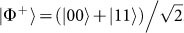. The photons interfere at the polarising beam splitters (PBS) as shown in Fig. 1. The PBS on the control (target) side is aligned to act in the basis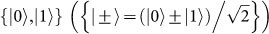. The photons are filtered spectrally and spatially using narrow-band filters (Δλ = 3 nm) and by coupling them into single-mode fibers. A coincidence detection of the ancilla qubits in detectors 3 and 4 in the state |–〉3|1〉4 signals a successful gate operation5,6,7.

The output photons of the first CNOT (modes I and II in Fig. 1) are then guided to the second CNOT. This gate is implemented in a destructive way, where a coincident measurement of the output photons signals the correct gate operation. The basic element of this destructive CNOT is a polarisation-dependent beam splitter (PDBS) which has a different transmission coefficient T for horizontally and vertically polarised light (TH = 1, TV = 1/3)5,6,7. If two vertically-polarised photons are reflected at this PDBS, they acquire a phase shift of π. Subsequent PDBSs with opposite splitting ratios equalise the output amplitudes. This setup, in combination with two half-wave plates (HWPs) (Fig. 3b) implements a destructive CNOT. The success of this second CNOT is determined by postselection on a coincidence detection in outputs 1 and 2.

Combining these photonic CNOT gates with local unitary operations allows us to implement a two-qubit quantum processor. In our setup, we implement these local unitary operations using quarter- and half-wave plates (QWP and HWP), which can be easily adjusted to program the quantum computer (Fig. 1 and 3b). Fig. 2 shows a sample run of our experiment; details about experimental parameters can be found in Methods and in the Supplementary Information (SI).

We use our two-qubit quantum processor to solve systems of linear equations, which, given a matrix A and a vector b, means finding the vector x such that Ax = b. If we rescale the vectors to ||b|| = ||x|| = 1 and represent them as quantum states |b〉 and |x〉, the task becomes finding |x〉 such that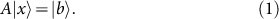The solution is |x〉 = A−1 |b〉/||A−1 |b〉||.

The quantum algorithm14 consists of three steps, described in Methods. There, we also discuss the simplest possible realisation of the algorithm, for a 2 × 2 matrix A and its reduction to the simplified circuit shown in Fig. 3a. This circuit depends on the eigenvalues, λ1 and λ2, of the matrix A, the unitary R that diagonalises it,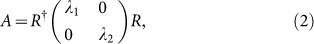and the input state |b〉.

We have implemented various instances of the algorithm, varying all the parameters in A, R and |b〉. The control and target qubits are prepared in the states R|b〉 and |1〉, respectively, absorbing the local operation R into the preparation of the input state. The matrix A can be modified by tuning the local operation R (in which case the eigenvalues stay the same) or by adapting the eigenvalues of the matrix, λ1 and λ2, which is done by adapting the local unitary operations before and after the second CNOT gate (see Fig. 3). Finally, the detection of the ancilla qubit in the state |1〉 announces a successful run of the algorithm and the preparation of the output qubit in the state |x〉. The success probability is (λ12)2. Because we are able to distinguish two eigenvalues, our method, although different and using logical fewer qubits, is as powerful as that of Cai et al15.

The output state is analysed using HWPs, QWPs and polarising beam splitters and a full state tomography is performed. Error estimates are obtained from a Monte Carlo routine assuming Poissonian counting statistics. These indicate a lower bound for the actual error that takes all the experimental imperfections into account.

Detailed results of a sample run of the algorithm are shown in Fig. 3. Additional results are presented in Fig. 4, where the input states and the eigenvalues of A are varied. It can be seen there that the performance of the algorithm depends on the input state R|b〉. To analyse this behaviour, we chose two different input states, |b1〉 = |1〉 and |b2〉 = |+〉 for each A, while keeping R equal to the identity matrix. We achieve fidelities of up to 0.981 ± 0.009 for |b1〉 and 0.832 ± 0.031 for |b2〉. The difference in fidelities arises due to the influence of higher-order emissions, as discussed in the SI. Data for additional choices of A, R and |b〉 are shown in the SI. The reported density matrices contain the raw measured data and have not been modified in the post processing.

Discussion

We present a two-qubit quantum circuit with two concatenated entangling gates acting on the same pair of photonic qubits and employ our system to implement the simplest case of the quantum algorithm for solving systems of linear equations. With demonstrating the consecutive application of two CNOT gates on the same pair of qubits, we have addressed a technological challenge in photonic quantum computation. This development is a step towards universal multi-qubit quantum processors using single photons as a versatile platform for computation and simulation.

We anticipate that increasing technological capabilities, including the implementation of more than two consecutive CNOTs, will allow the extension of the algorithm both to larger systems and to more precise phase estimation. Eventually, this will allow not only solving larger systems of linear equations, but also equally important algorithms that use it as a subroutine, including quantum algorithms for solving nonlinear differential equations21 and quantum data fitting22.

Methods

Theory

We assume, without loss of generality14, that A is an N × N Hermitian matrix with eigenbasis {|uj〉} and eigenvalues {λj}, rescaled so that 0 < λj < 1. If |b〉 is expanded as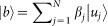, we aim to prepare, up to normalization,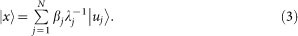The quantum algorithm14 consists of three steps. The most general circuit involves phase estimation, a controlled Ry rotation and a reverse phase estimation. Our work differs from the original proposal14 in several modifications that are needed to implement the algorithm with a limited number of qubits. Here, we briefly outline the algorithm while noting our modifications.

The first step, phase estimation, is a general procedure for decomposing quantum states into a particular basis1,23. It requires an additional “eigenvalue” register of m qubits, each initialised to |0〉. Phase estimation transforms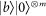into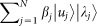, where the eigenvalues |λj〉 are stored in the eigenvalue register to a precision of m bits (binary digits).

We restrict ourselves to the simplest case, which involves one state qubit and one eigenvalue qubit. The single state qubit implies that |b〉 is a two-vector and A a 2 × 2 matrix, while the single eigenvalue qubit means that only a single bit of the eigenvalues is computed by the phase estimation. This means that for the algorithm to work perfectly, it must be possible to distinguish the two eigenvalues with reference to a single bit. Consequently, we choose the two eigenvalues to be of the form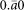and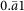, whereis a sequence of bits. The position of the differing bit is what governs the choice of the phase estimation unitary U. In particular, if we wish to read out the nth bit, we must choose U = exp (2πi 2n−1A). We note that choosing eigenvalues that could not be distinguished using a single bit would lead to additional rounding errors, which would, in principle, be avoidable with a larger quantum computer.

The two-qubit phase estimation transforms the state and eigenvalue qubits, initialised to |bS|0〉E to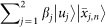, where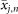is the nth bit of λj. Each eigenstate in the state register is now entangled to the nth bit of its eigenvalue.

The second step is the nonunitary map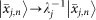. For this, we introduce an additional “ancilla” qubit initialised to |1〉 and controlled on the value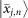in the eigenvalue register, we implement a controlled-Ryj) = exp(−jσy/2) rotation on the ancilla qubit, where σy is the Pauli matrix. With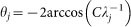(where C ≤ minjj|), the overall state becomes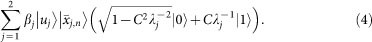The third step is to run phase estimation in reverse to uncompute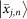, giving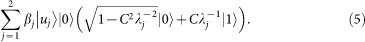We measure the ancilla and if we observe a 1 we will have prepared |x〉 in the state register. If we know the eigenvalues, we can maximise the success probability by choosing the largest possible C, C = minjj|.

The procedure described above involves three qubits, one each for the state, eigenvalue and ancilla registers. The corresponding circuit is shown in Fig. 5, which also describes how it can be simplified to the final circuit shown in Fig. 3a.

Experiment

For the creation of entangled photon pairs, a mode-locked Coherent Mira HP Ti:Sa oscillator is pumped by a Coherent Inc. Verdi V-10 laser. The pulsed-laser output (τ = 200 fs, λ = 789 nm, 76 MHz) is frequency-doubled using a 2 mm-thick lithium triborate (LBO) crystal, resulting in UV pulses of 0.75 W cw average. We achieve a stable source of UV pulses by translating the LBO to avoid optical damage to the anti-reflection coating of the crystal. Our detectors have quantum efficiencies of 0.4 and dark count rates between 500 and 3500 counts per second. In our experiment, typical visibilities (fidelities) of the emitted Bell pairs of about 0.9 (0.8) and higher-order emissions degrade the quality of our gate operations. The back-reflecting mirror is continuously moved back-and-forth to avoid any phase correlations between of the signal and the noise originating from higher-order photon emissions. Additionally, imperfect visibilities on the order of 0.85 to 0.9 of the quantum interference (caused by partial distinguishability of the photons) at the PBSs in the first gate and the PDBS in the second gate contribute to errors. The measured production rate of two-fold coincidences is 18000 s−1 in the forward direction and 10000 s−1 in the backward direction (measured after the first gate). The total fourfold-coincidence rate is 1700 h−1 (55 h−1) after the first gate (both gates).

Change history

• 12 May 2017

A correction has been published and is appended to both the HTML and PDF versions of this paper. The error has been fixed in the paper.

• 30 June 2015

The HTML version of this Article previously published incorrectly listed Philip Walther and not Stefanie Barz, as corresponding author. This has now been corrected in the HTML; the PDF version of the paper was correct from the time of publication.

References

1. Nielsen, M. A. & Chuang, I. L. Quantum Computation and Quantum Information (Cambridge University Press, Cambridge, 2000).

2. Hanneke, D. et al. Realization of a programmable two-qubit quantum processor. Nat. Phys. 6, 13–16 (2009).

3. Knill, E., Laflamme, R. & Milburn, G. J. A scheme for efficient quantum computation with linear optics. Nature 409, 46–52 (2001).

4. O'Brien, J. L., Pryde, G. J., White, A. G., Ralph, T. C. & Branning, D. Demonstration of an all-optical quantum controlled-NOT gate. Nature 426, 264–267 (2003).

5. Langford, N. K. et al. Demonstration of a simple entangling optical gate and its use in Bell-state analysis. Phys. Rev. Lett. 95, 210504 (2005).

6. Kiesel, N., Schmid, C., Weber, U., Ursin, R. & Weinfurter, H. Linear optics controlled-phase gate made simple. Phys. Rev. Lett. 95, 210505 (2005).

7. Okamoto, R., Hofmann, H. F., Takeuchi, S. & Sasaki, K. Demonstration of an optical quantum controlled-NOT gate without path interference. Phys. Rev. Lett. 95, 210506 (2005).

8. Zhou, X. et al. Adding control to arbitrary unknown quantum operations. Nat. Comm. 2, 413 (2011).

9. Crespi, A. et al. Integrated photonic quantum gates for polarization qubits. Nat. Comm. 2, 566 (2011).

10. Sansoni, L. et al. Polarization entangled state measurement on a chip. Phys. Rev. Lett. 105, 200503 (2010).

11. Martín-López, E. et al. Experimental realization of Shor's quantum factoring algorithm using qubit recycling. Nat. Photon. 6, 773–776 (2012).

12. Pittman, T., Jacobs, B. & Franson, J. Probabilistic quantum logic operations using polarizing beam splitters. Phys. Rev. A 64, 062311 (2001).

13. Gasparoni, S., Pan, J.-W., Walther, P., Rudolph, T. & Zeilinger, A. Realization of a photonic controlled-NOT gate sufficient for quantum computation. Phys. Rev. Lett. 93, 020504 (2004).

14. Harrow, A., Hassidim, A. & Lloyd, S. Quantum algorithm for linear systems of equations. Phys. Rev. Lett. 103, 150502 (2009).

15. Cai, X.-D. et al. Experimental quantum computing to solve systems of linear equations. Phys. Rev. Lett. 110, 230501 (2013).

16. Cao, Y., Daskin, A., Frankel, S. & Kais, S. Quantum circuit design for solving linear systems of equations. Mol. Phys. 110, 1675–1680 (2012).

17. Pan, J. et al. Experimental realization of quantum algorithm for solving linear systems of equations. Phys. Rev. A 89, 022313 (2013).

18. Kok, P. et al. Linear optical quantum computing with photonic qubits. Rev. Mod. Phys. 79, 135–174 (2007).

19. O'Brien, J. & Akira Furusawa, J. Vučković. Photonic quantum technologies. Nat. Photon. 3, 687–695 (2009).

20. Kwiat, P. G., Waks, E., White, A. G., Appelbaum, I. & Eberhard, P. H. Ultrabright source of polarization-entangled photons. Phys. Rev. A 60, R773–R776 (1999).

21. Leyton, S. K. & Osborne, T. J. A quantum algorithm to solve nonlinear differential equations. arXiv, 0812.4423 (2008).

22. Wiebe, N., Braun, D. & Lloyd, S. Quantum algorithm for data fitting. Phys. Rev. Lett. 109, 050505 (2012).

23. Kitaev, A. Quantum computations: algorithms and error correction. Russ. Math. Surv. 52, 1191–1249 (1997).

24. Lanyon, B. P. et al. Simplifying quantum logic using higher-dimensional hilbert spaces. Nat. Phys. 5, 134–140 (2009).

25. Lanyon, B. et al. Towards quantum chemistry on a quantum computer. Nat. Chem. 2, 106–111 (2010).

26. Zhou, X.-Q., Kalasuwan, P., Ralph, T. C. & O'Brien, J. L. Calculating unknown eigenvalues with a quantum algorithm. Nat. Photon. 7, 223–228 (2013).

Acknowledgements

We thank Frank Verstraete for valuable discussions and Thomas Lindner for assistance in the laboratory. I.K. was supported by a UQ Postdoctoral Research Fellowship and the ARC Centres of Excellence for Engineered Quantum Systems (CE110001013) and Quantum Computation and Communication Technology (CE110001027). A.A.G. thanks the Hughes Research Laboratory (M1144-201167-DS), Air Force Research Office (FA9550-12-1-0046 and 10323836-SUB) and the Corning, Sloan and Dreyfus foundations for their support. P.W. acknowledges support from the European Commission, Q-ESSENCE (No. 248095), QUILMI (No. 295293), EQUAM (No. 323714), PICQUE (No. 608062), GRASP (No. 613024) and the ERA-Net CHISTERA project QUASAR, the John Templeton Foundation, the Vienna Center for Quantum Science and Technology (VCQ), the Austrian Nano-initiative NAP Platon, the Austrian Science Fund (FWF) through the SFB FoQuS (F4006-N16), START (Y585-N20) and the doctoral programme CoQuS, the Vienna Science and Technology Fund (WWTF, grant ICT12-041) and the United States Air Force Office of Scientific Research (FA8655-11-1-3004).

Author information

Authors

Contributions

S.B., M.R. and Y.O.L. designed and implemented the experiment. S.B. and M.R. collected and analysed the data. I.K. and A.A.G. provided the theoretical proposal and I.K. and B.D. provided the theoretical analysis. A.A.G. and P.W. supervised the project. All authors wrote and edited the paper.

Ethics declarations

Competing interests

The authors declare no competing financial interests.

Electronic supplementary material

Supplementary Information

Supplementary Information: A two-qubit photonic quantum processor and its application to solving systems of linear equations

Rights and permissions

Reprints and Permissions

• Fusing the single-excitation subspace with $${\mathbb C}^{2^n}$$

• Michael R. Geller

Scientific Reports (2021)

• Simulation of Multiple-Quantum NMR Dynamics of Spin Dimer on Quantum Computer

• S. I. Doronin
• E. B. Fel’dman
• A. I. Zenchuk

Applied Magnetic Resonance (2021)

• Solving systems of linear algebraic equations via unitary transformations on quantum processor of IBM Quantum Experience

• S. I. Doronin
• E. B. Fel’dman
• A. I. Zenchuk

Quantum Information Processing (2020)

• Hybrid classical-quantum linear solver using Noisy Intermediate-Scale Quantum machines

• Chih-Chieh Chen
• Shiue-Yuan Shiau
• Yuh-Renn Wu

Scientific Reports (2019)

• Quantum Algorithm Design: Techniques and Applications

• Changpeng Shao
• Yang Li
• Hongbo Li

Journal of Systems Science and Complexity (2019)